Courses
Courses for Kids
Free study material
Free LIVE classes
More

# NCERT Solutions for Class 3 Maths Chapter 9 - How Many TimesLIVE
Join Vedantu’s FREE Mastercalss

## NCERT Solutions for Class 3 Maths Chapter 9 - How Many Times

NCERT Solutions for Class 3 Maths Chapter 9 provides a comprehensive study guide for understanding the related concepts of multiplication and repeated addition. The solutions of the sums given in the chapter How Many Times are prepared by our highly experienced faculty. They have maintained a comprehensive approach towards problem-solving in every sum. Every sum in the NCERT Solutions of Class 3 Maths Chapter 9, is solved in a step-by-step manner and is followed by a detailed explanation. Download How Many Times Class 3 NCERT Solutions for free from Vedantu, and practice them to fetch good scores in the exams.

Last updated date: 19th Sep 2023
Total views: 460.2k
Views today: 10.60k

## Access NCERT Solutions for Class 3 Mathematics Chapter 9 – How Many Times

1. How Many Spiders? ________

One spider has _____ legs.

In all, spider legs are 3 times _______

Or $\_\_\_\_ + \_\_\_\_\_ + \_\_\_\_ = \_\_\_\_\_$

Or $3 \times \_\_\_\_ = \_\_\_\_$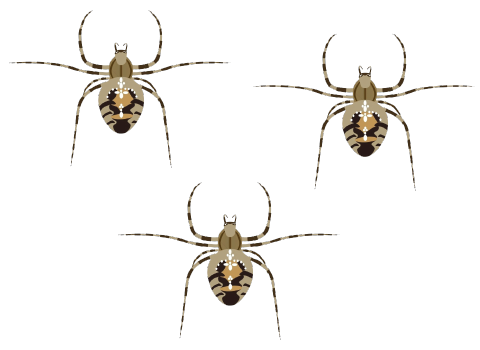Ans: As we can see that there are three spiders. Each spider has 8 legs.

In all, spiders are 3 times 8.

Or $8 + 8 + 8 = 24$

Or $3 \times 8 = 24$

2. Do you know this leggy fellow?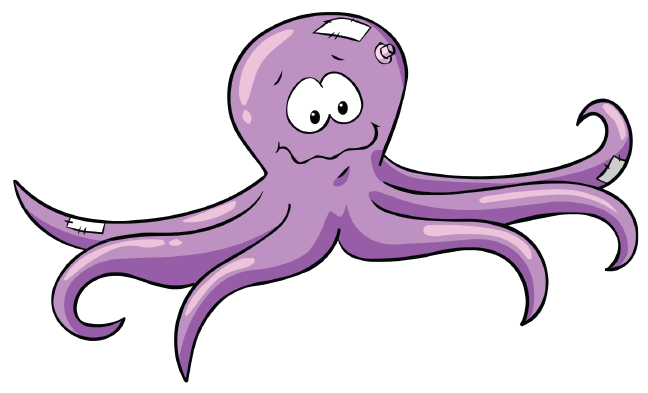This is an octopus. It lives in the sea. It also has 8 legs.

So how many legs altogether do 5 octopuses have?

$\_\_\_\_ + \_\_\_\_\_ + \_\_\_\_ + \_\_\_\_ + \_\_\_\_ = \_\_\_\_\_$

Or $5{\text{ times}}\_\_\_\_ = \_\_\_\_$

Or $5 \times \_\_\_\_ = \_\_\_\_$

Ans: In the figure, we can see that one octopus has 8 legs.

$8 + 8 + 8 + 8 + 8 = 40$

Or $5{\text{ times }}8 = 40$

Or $5 \times 8 = 40$

3. In the same way, how many bottles are these?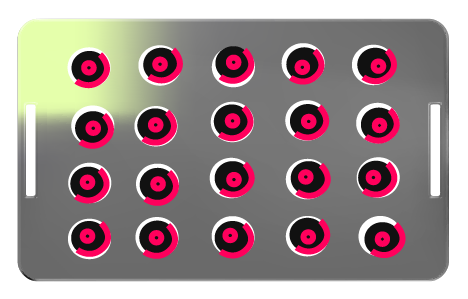$\_\_\_\_{\text{ times }}\_\_\_\_ = \_\_\_\_{\text{ bottles}}$

Ans: There are 5 rows and each row has 4 bottles.

${\text{5 times }}4 = 20{\text{ bottles}}{\text{.}}$

4. How Many Eggs?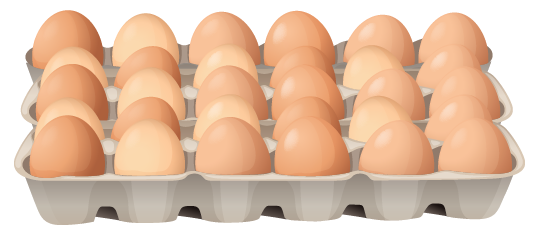$\_\_\_\_{\text{ times }}\_\_\_\_ = \_\_\_\_{\text{ eggs}}$

Ans: There are 5 rows and each row has 6 bottles.

${\text{5 times 6}} = 30{\text{ egges}}{\text{.}}$

5. Rewrite using the $+$ sign.

$2 \times 5$ is 2 times 2 or $5 + 5$

$4 \times 18$ is 4 times $\_\_\_\_\_$ or $\_\_\_\_\_ + \_\_\_\_\_ + \_\_\_\_\_ + \_\_\_\_\_$

$3 \times 20$ is $\_\_\_\_\_$ times $\_\_\_\_\_$ or $\_\_\_\_\_ + \_\_\_\_\_ + \_\_\_\_\_$

$8 \times 9$ is $\_\_\_\_\_$ times $\_\_\_\_\_$ or $\_\_\_\_\_ + \_\_\_\_\_ + \_\_\_\_\_ + \_\_\_\_\_ + \_\_\_\_\_ + \_\_\_\_\_ + \_\_\_\_\_ + \_\_\_\_\_$

Ans:

$2 \times 5$ is 2 times 2 or $5 + 5$

$4 \times 18$ is 4 times 18 or $18 + 18 + 18 + 18$

$3 \times 20$ is 3 times 20 or $20 + 20 + 20$

$8 \times 9$ is 8 times 9 or $9 + 9 + 9 + 9 + 9 + 9 + 9 + 9$

6. Tell How Many Times!

 $9 + 9 + 9 + 9 + 9 + 9$ $= 6 \times 9$ $= 54$ $4 + 4 + 4 + 4 + 4$ $= 5 \times 4$ $= 20$ $8 + 8 + 8$ $= \_\_\_ \times 8$ $= \_\_\_$ $3 + 3 + 3 + 3 + 3$ $= 5 \times \_\_\_$ $= \_\_\_$ $30 + 30 + 30$ $= \_\_\_ \times \_\_\_$ $= \_\_\_$ $7 + 7 + 7 + 7 + 7 + 7$ $= \_\_\_ \times \_\_\_$ $= \_\_\_$ $12 + 12 + 12 + 12$ $= \_\_\_ \times 12$ $= \_\_\_$ $6 + 6 + 6$ $= \_\_\_ \times \_\_\_$ $= \_\_\_$ $10 + 10 + 10 + 10$ $= \_\_\_ \times \_\_\_$ $= \_\_\_$ $2 + 2 + 2 + 2 + 2$ $= \_\_\_ \times \_\_\_$ $= \_\_\_$ $6 + 6 + 6 + 6 + 6 + 6 + 6$ $= \_\_\_ \times \_\_\_$ $= \_\_\_$

Ans:

 $9 + 9 + 9 + 9 + 9 + 9$ $= 6 \times 9$ $= 54$ $4 + 4 + 4 + 4 + 4$ $= 5 \times 4$ $= 20$ $8 + 8 + 8$ $= 3 \times 8$ $= 24$ $3 + 3 + 3 + 3 + 3$ $= 5 \times 3$ $= 15$ $30 + 30 + 30$ $= 3 \times 30$ $= 90$ $7 + 7 + 7 + 7 + 7 + 7$ $= 6 \times 7$ $= 42$ $12 + 12 + 12 + 12$ $= 4 \times 12$ $= 48$ $6 + 6 + 6$ $= 3 \times 6$ $= 18$ $10 + 10 + 10 + 10$ $= 4 \times 10$ $= 40$ $2 + 2 + 2 + 2 + 2$ $= 5 \times 2$ $= 10$ $6 + 6 + 6 + 6 + 6 + 6 + 6$ $= 7 \times 6$ $= 42$

7. Ramu bought 4 packets of biscuits. Each packet has 4 biscuits. How many biscuits did Ramu buy?

Ans: Given,

The number of biscuits Ramu bought is 4.

The number of biscuits each packet has 4.

Number of biscuits in 4 packets

$= 4 + 4 + 4 + 4 = 4 \times 4 = 16$

Thus, Ramu bought 16 biscuits all total.

8. There are 12 desks in a classroom. Each desk has 4 legs. What is the total number of legs of the desks?

Ans: Given

The number of desks in a classroom is 12.

The number of legs on each desk has 4.

Number of legs of all 12 desks in a classroom

$= 12 \times 4 = 48.$

Thus, there are a total, 48 legs of the desk in the classroom.

9. Sabiha brought home 3 bunches of flowers. Each bunch has 4 flowers. How many flowers were there?

Ans: Given,

The number of bunches of flowers Sabiha bought is 3.

Number of flowers in each bunch is 4.

Number of flowers in 3 bunches

$= 3 \times 4 = 12$

Thus, there are 12 flowers.

10. One rail coach has 8 wheels. How many wheels in all in 6 coaches?

Ans: Given,

The number of coaches is 6.

The number of wheels in each coach is 8.

Number of wheels in 6 coaches

$= 6 \times 8 = 48$

Thus, there are 48 wheels in all 6 coaches.

11. How Many Times 2?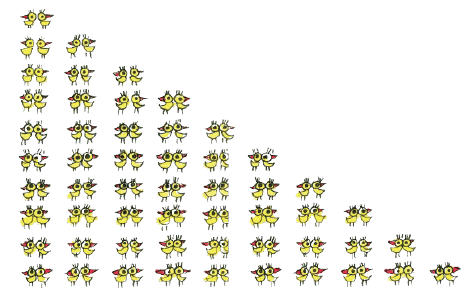${\text{1 times }}2$ ${\text{is }}2$ ${\text{or 1}} \times {\text{2}}$ $= 2$ ${\text{2 times }}2$ ${\text{is 4}}$ ${\text{or 2}} \times {\text{2}}$ $= 4$ ${\text{3 times }}2$ ${\text{is 6}}$ ${\text{or 3}} \times {\text{2}}$ $= 6$ ${\text{4 times }}2$ ${\text{is }}\_\_\_$ ${\text{or 4}} \times {\text{2}}$ $= \_\_\_$ ${\text{5 times }}2$ ${\text{is }}\_\_\_$ ${\text{or 5}} \times {\text{2}}$ $= \_\_\_$ $6{\text{ times }}2$ ${\text{is }}\_\_\_$ ${\text{or 6}} \times {\text{2}}$ $= \_\_\_$ $\_\_\_\_\_{\text{ times }}2$ ${\text{is }}\_\_\_$ ${\text{or \_\_\_}} \times {\text{2}}$ $= \_\_\_$ $\_\_\_\_\_{\text{ times \_\_\_\_}}$ ${\text{is }}\_\_\_$ ${\text{or 8}} \times {\text{2}}$ $= \_\_\_$ $\_\_\_\_\_{\text{ times \_\_\_\_}}$ ${\text{is }}\_\_\_$ ${\text{or 9}} \times {\text{2}}$ $= \_\_\_$ $\_\_\_\_\_{\text{ times \_\_\_\_}}$ ${\text{is }}\_\_\_$ ${\text{or 10}} \times {\text{2}}$ $= \_\_\_$

Ans:

 ${\text{1 times }}2$ ${\text{is }}2$ ${\text{or 1}} \times {\text{2}}$ $= 2$ ${\text{2 times }}2$ ${\text{is 4}}$ ${\text{or 2}} \times {\text{2}}$ $= 4$ ${\text{3 times }}2$ ${\text{is 6}}$ ${\text{or 3}} \times {\text{2}}$ $= 6$ ${\text{4 times }}2$ ${\text{is }}8$ ${\text{or 4}} \times {\text{2}}$ $= 8$ ${\text{5 times }}2$ ${\text{is 10}}$ ${\text{or 5}} \times {\text{2}}$ $= 10$ $6{\text{ times }}2$ ${\text{is 12}}$ ${\text{or 6}} \times {\text{2}}$ $= 12$ ${\text{7 times }}2$ ${\text{is 14}}$ ${\text{or 7}} \times {\text{2}}$ $= 14$ ${\text{8 times }}2$ ${\text{is 16}}$ ${\text{or 8}} \times {\text{2}}$ $= 16$ ${\text{9 times }}2$ ${\text{is 18}}$ ${\text{or 9}} \times {\text{2}}$ $= 18$ ${\text{10 times }}2$ ${\text{is 20}}$ ${\text{or 10}} \times {\text{2}}$ $= 20$

12. Jump with Me

Tarru Frog jumps 3 steps each time. Which numbers will Tarru touch?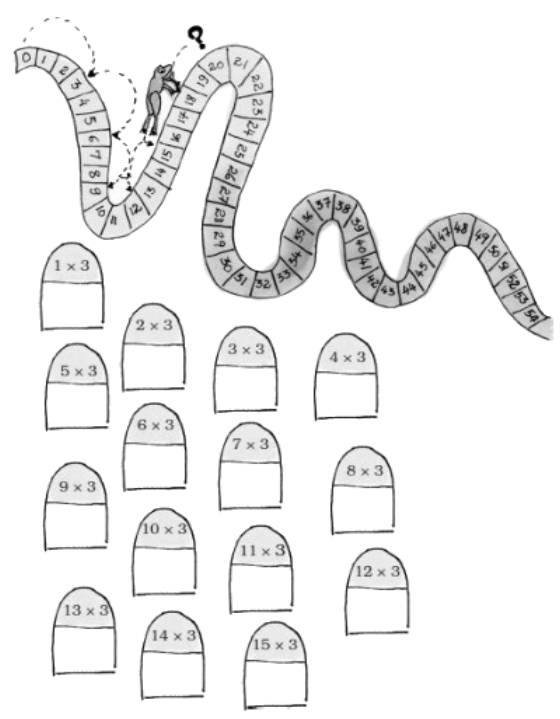Ans: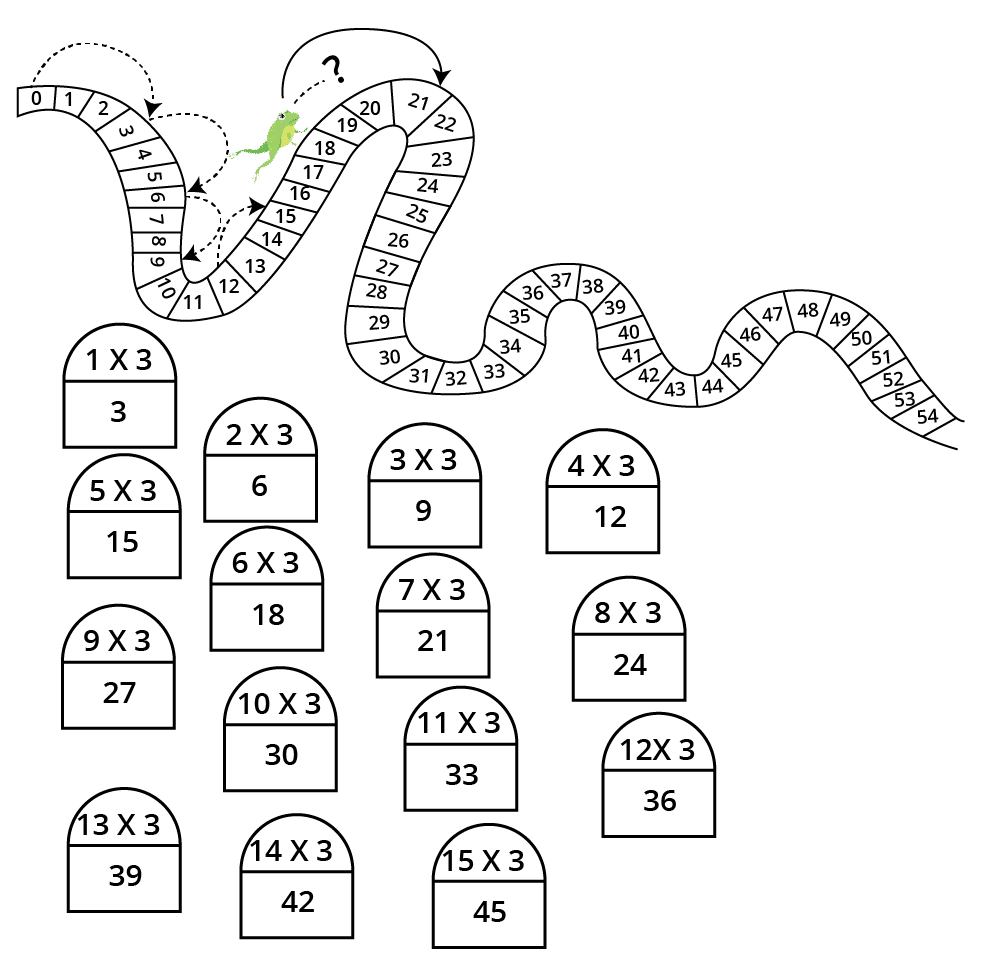13. Show Jumps with 4 Steps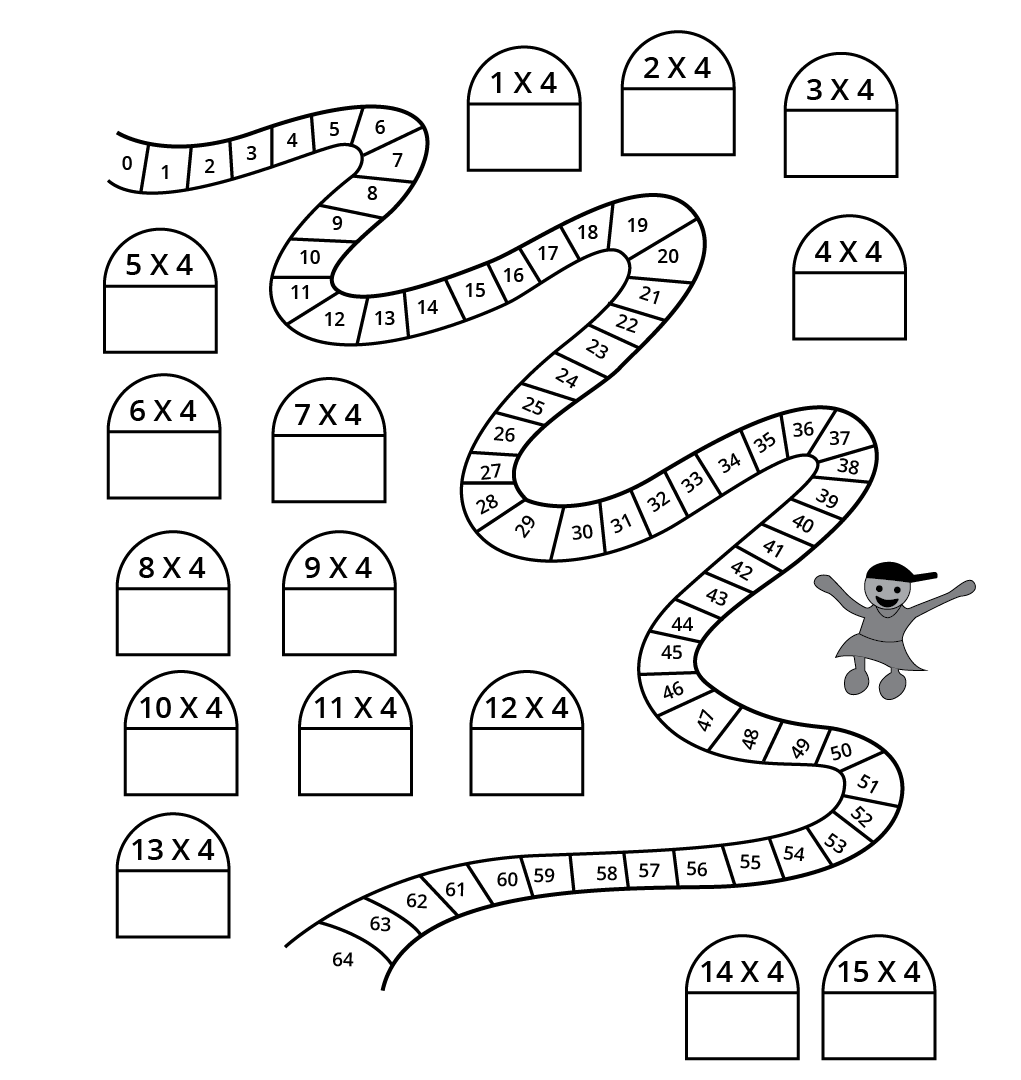Ans: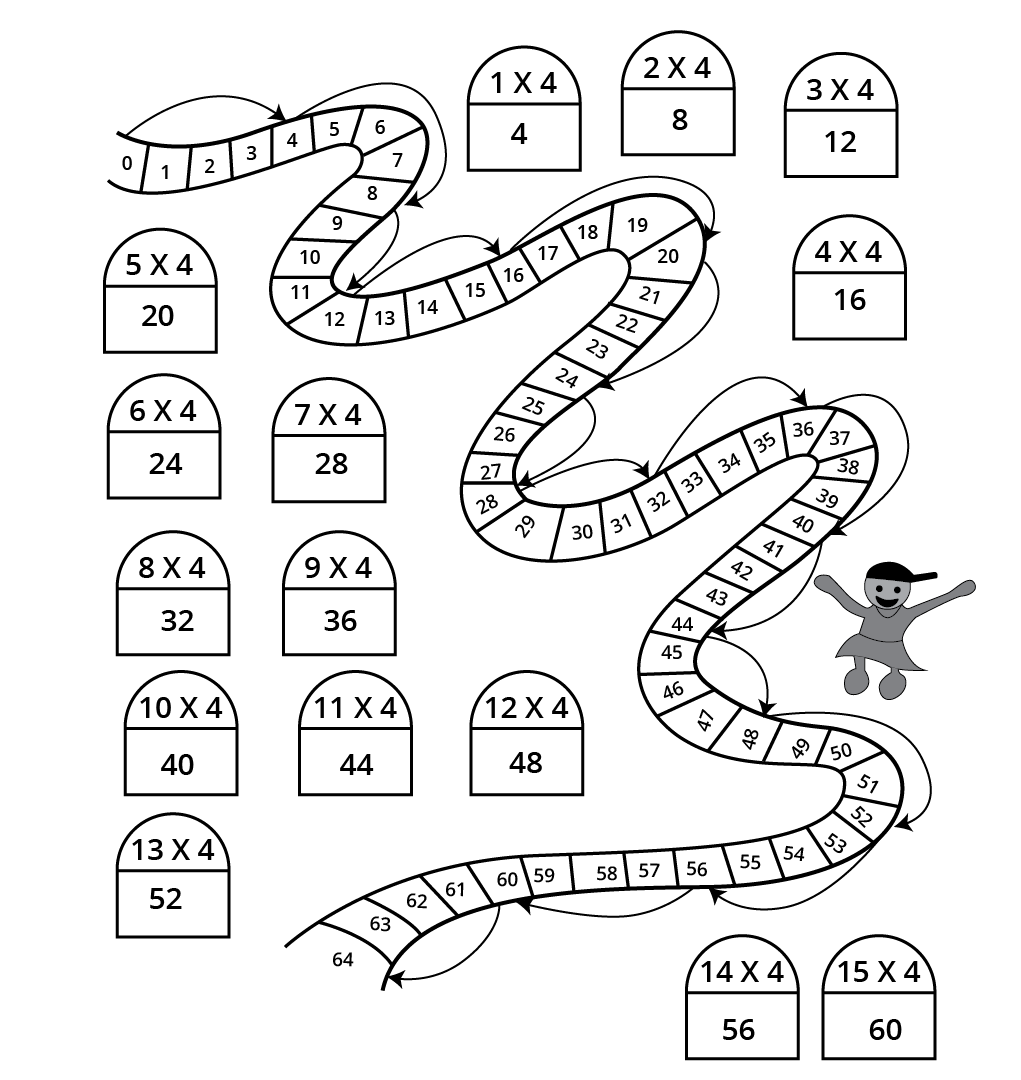14. Try Jumps with Seven Steps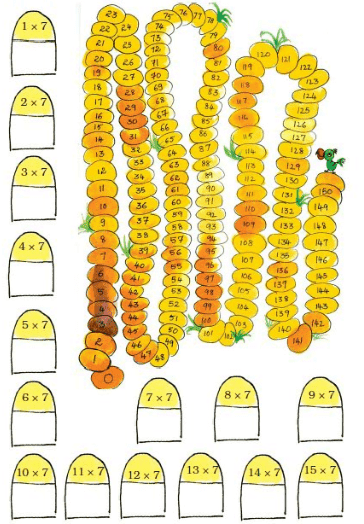Ans: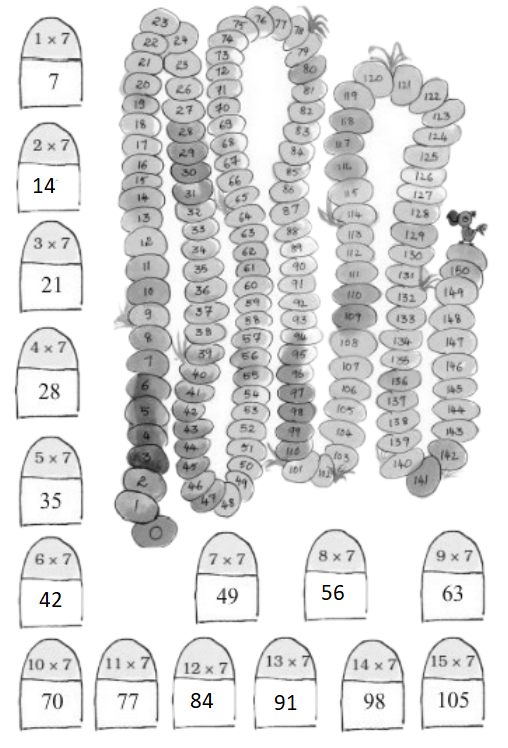15. Let's Try Making a 2 Times Table with Sticks: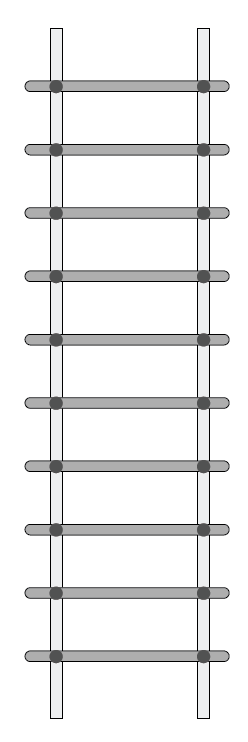$1 \times 2 = 2$

$2 \times 2 = 4$

$3 \times 2 = 6$

$4 \times 2 = \_\_\_$

$5 \times 2 = \_\_\_$

$6 \times 2 = \_\_\_$

$7 \times 2 = \_\_\_$

$8 \times 2 = \_\_\_$

$9 \times 2 = \_\_\_$

$10 \times 2 = \_\_\_$

Ans:

$1 \times 2 = 2$

$2 \times 2 = 4$

$3 \times 2 = 6$

$4 \times 2 = 8$

$5 \times 2 = 10$

$6 \times 2 = 12$

$7 \times 2 = 14$

$8 \times 2 = 16$

$9 \times 2 = 18$

$10 \times 2 = 20$

16. Now Draw Sticks to Make the Multiplication Table of 6: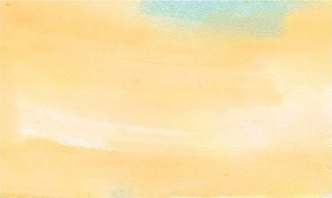Ans: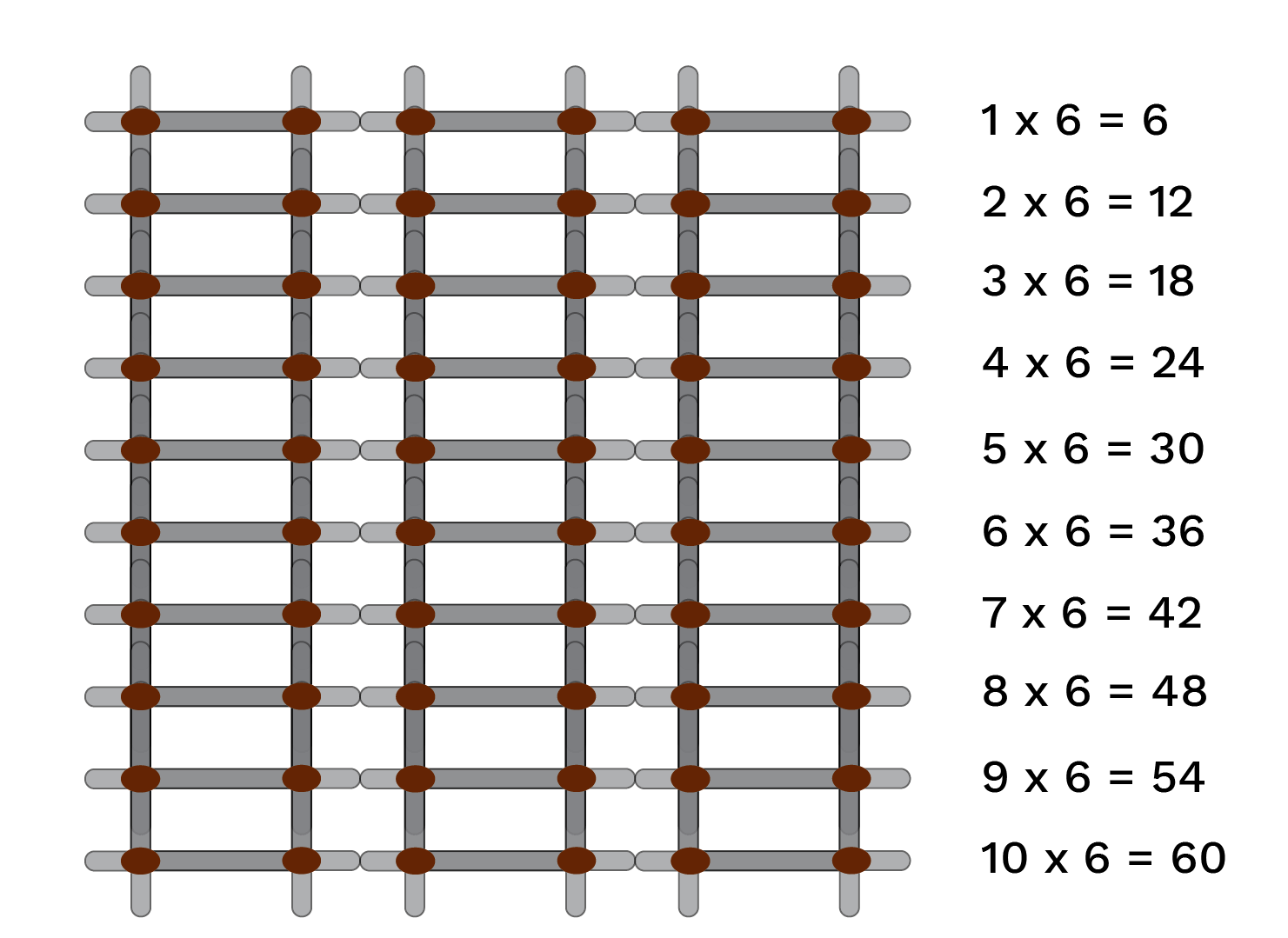17. Shopping with Tables How much do these things cost?

a) 4 toffees cost ________ rupees.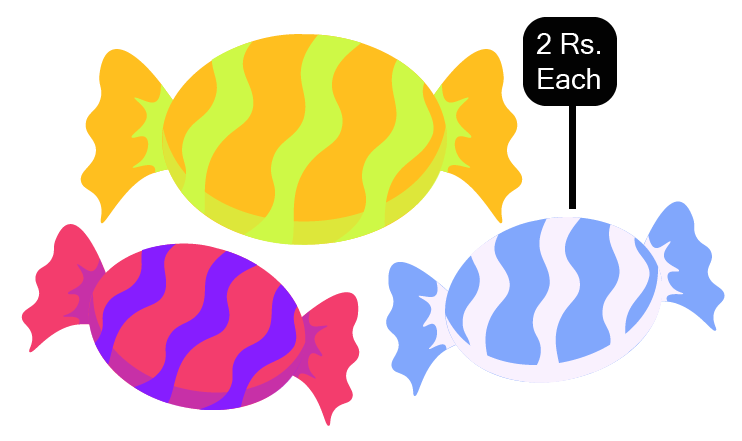Ans: Here we can see that the number of toffees is 4.

We know that price of each toffee is rupees 2.

Hence, the price of all the 4 toffees are

$= 4 \times 2 = Rs.\,8$

b) 3 pencil boxes cost ________ rupees. 10 pencil boxes cost ________ rupees.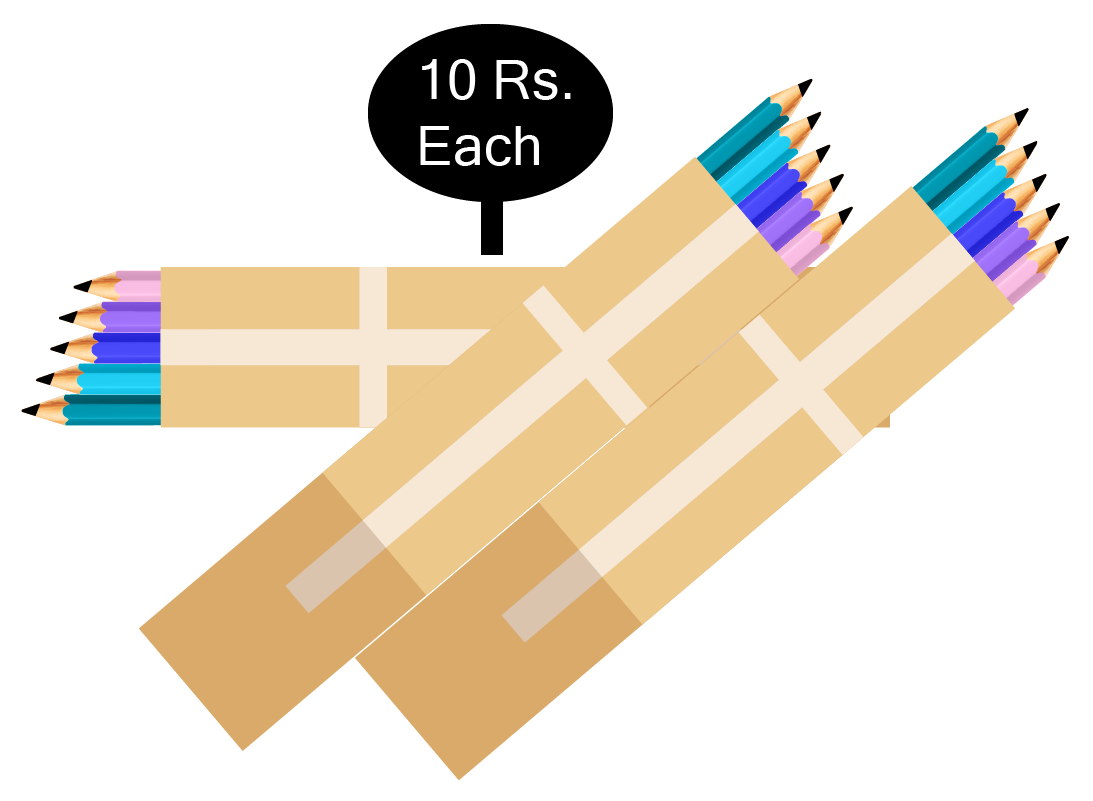Ans: We can see that each pencil box costs rupees 10 and here there are 3 pencil boxes.

Hence, 3 pencil box costs $3 \times 10$, that is rupees 30.

The 10 pencil boxes cost $10 \times 10$, that is rupees 100.

c) 9 balloons cost ________ rupees.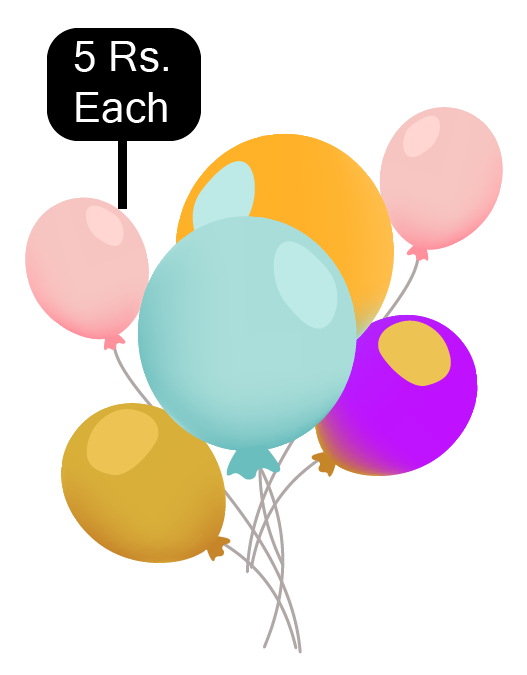Ans: Each balloon’s price is 5 rupees.

the price of 9 balloons is 9 times 5 rupees.

$\Rightarrow 9 \times 5 = Rs.\,45$

d) 5 toys cost _________ rupees.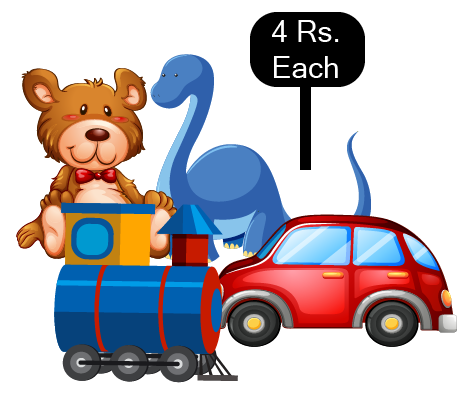Ans: Each toys price is 4 rupees.

the price of 5 toys is 5 times 4 rupees.

$\Rightarrow 5 \times 4 = Rs.\,20$

e) 7 face masks cost ________ rupees.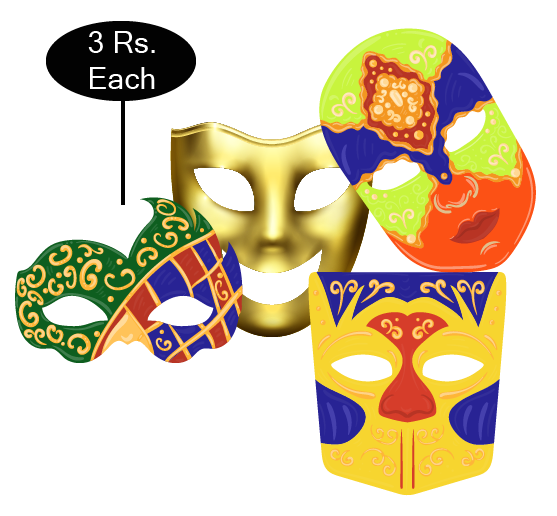Ans: Each face mask price is 3 rupees.

the price of 7 face masks is 7 times 3 rupees.

$\Rightarrow 7 \times 3 = Rs.\,21$

18: Practice Time Complete the following:

$2 \times 7 = \_\_\_$

$4 \times 9 = \_\_\_$

$5 \times 8 = \_\_\_$

$10 \times 6 = \_\_\_$

$5 \times 9 = \_\_\_$

$3 \times 9 = \_\_\_$

$5 \times 2 = \_\_\_$

$3 \times 10 = \_\_\_$

$2 \times 8 = \_\_\_$

$10 \times 8 = \_\_\_$

Ans:

$2 \times 7 = 14$

$4 \times 9 = 36$

$5 \times 8 = 40$

$10 \times 6 = 60$

$5 \times 9 = 45$

$3 \times 9 = 27$

$5 \times 2 = 10$

$3 \times 10 = 30$

$2 \times 8 = 16$

$10 \times 8 = 80$

19. Look at the Patterns and Complete them.

$3,\;6\;,9,\_\_\_,\_\_\_,\_\_\_.$

$2,\;4,\;6,\_\_\_,\_\_\_,\_\_\_.$

$10,\;20,\;30,\_\_\_,\_\_\_,\_\_\_.$

$4,\;8,\;12,\_\_\_,\_\_\_,\_\_\_.$

$5,\;10,\;15,\_\_\_,\_\_\_,\_\_\_.$

$30,\;60,\;90,\_\_\_,\_\_\_,\_\_\_.$

Ans:

$3,\;6,\;9,\;12,\;15,\;18$

$2,\;4,\;6,\;8,\;10,\;12$

$10,\;20,\;30,\;40,\;50,\;60$

$4,\;8,\;12,\;16,\;20,\;24$

$5,\;10,\;15,\;20,\;25,\;30$

$30,\;60,\;90,\;120,\;150,\;180$

20. Complete the multiplication tree

Ans: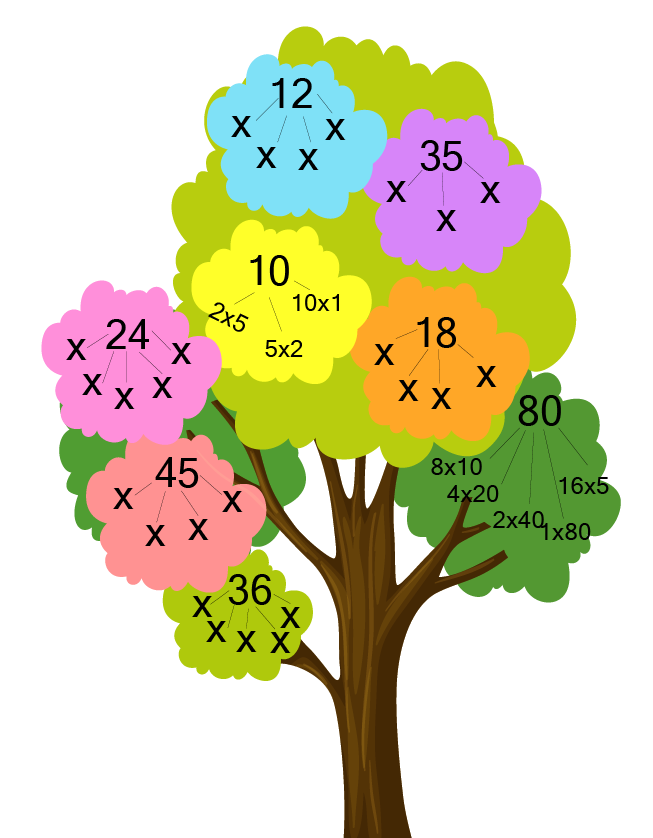Ans: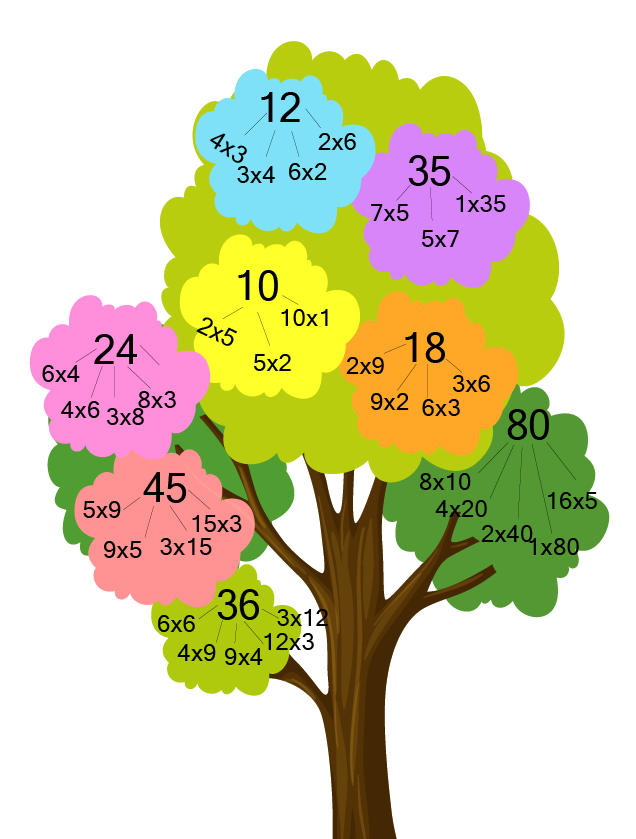21. How many in all?

a) A shirt has 5 buttons. How many buttons would 3 shirts have?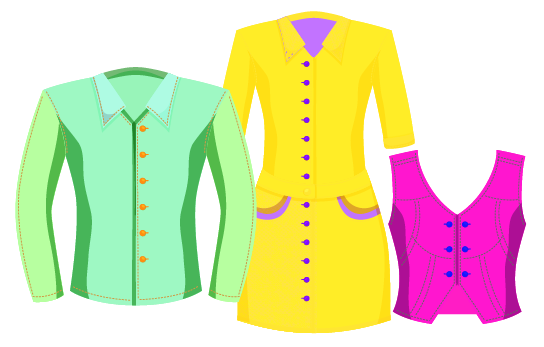Ans: The number of shirts is 3.

The number of buttons in each shirt is 5.

Hence the number of buttons in 3 shirts

$\Rightarrow 3 \times 5 = 15$

b) There are four fans. Each fan has 3 blades. What is the total number of blades in all?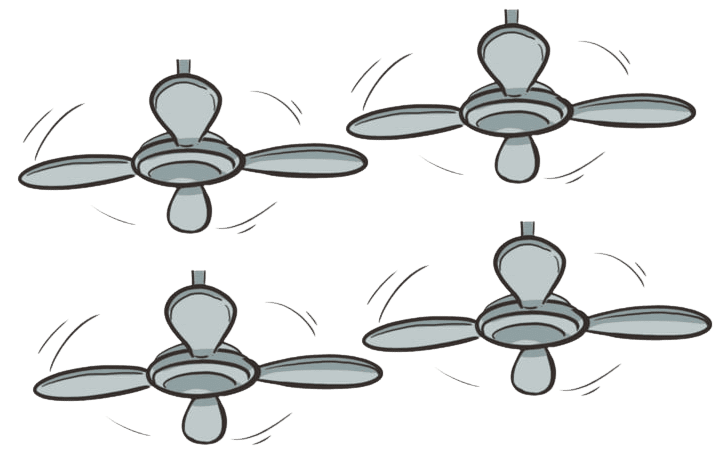Ans: The number of fans is 4.

The number of blades in each fan is 3.

Hence the number of blades in 4 fans

$\Rightarrow 4 \times 3 = 12$

c) A box contains 6 apples. How many apples in all will seven boxes have?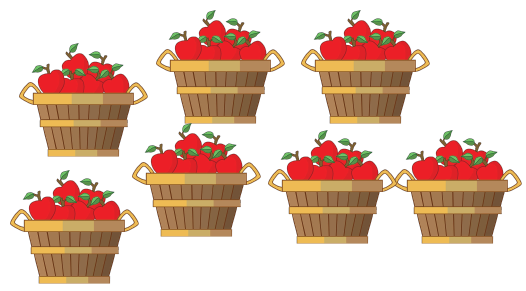Ans: The number of boxes is 7.

The number of apples in each box is 6.

Hence the number of apples in 7 boxes

$\Rightarrow 7 \times 6 = 42$

d) How many corners would 4 triangles have?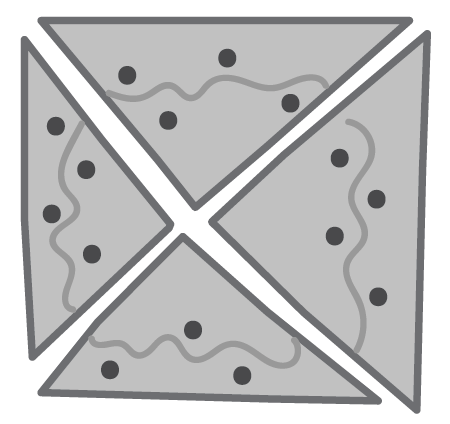Ans: The number of triangles is 4.

The number of corners in each triangle is 3.

Hence the number of corners in 4 triangles

$\Rightarrow 4 \times 3 = 12$

22.Some Multiplication Fats:

$8 \times 3 = \_\_\_$

$3 \times \_\_\_ = \_\_\_$

$\_\_\_\, \times \_\_\_ = 42$

$5 \times \_\_\_ = 40$

$\_\_\_ \times \_\_\_ = 54$

$5 \times \_\_\_ = 357$

$\_\_\_ \times 6 = 36$

$10 \times \_\_\_ = \_\_\_$

$\_\_\_ \times 9 = 36$

$\_\_\_ \times 7 = 28$

Ans:

$8 \times 3 = 24$

$3 \times 7 = 21$

$7 \times 6 = 42$

$5 \times 8 = 40$

$6 \times 9 = 54$

$5 \times 7 = 35$

$6 \times 6 = 36$

$10 \times 8 = 80$

$4 \times 9 = 36$

$4 \times 7 = 28$

23. Multiplication Table of 1

 One time one is $1 \times 1 =$ 1 Two time one is $2 \times 1 =$ 2 Three time one is $\_\_\_ \times \_\_\_$ $= \_\_\_$ Four time one is $\_\_\_ \times \_\_\_$ $= \_\_\_$ $\_\_\_$ time one is $\_\_\_ \times \_\_\_$ $= \_\_\_$ $\_\_\_$ time one is $\_\_\_ \times \_\_\_$ $= \_\_\_$ $\_\_\_$ time one is $\_\_\_ \times \_\_\_$ $= \_\_\_$ $\_\_\_$ time one is $\_\_\_ \times \_\_\_$ $= \_\_\_$

Ans:

 One time one is $1 \times 1 =$ 1 Two time one is $2 \times 1 =$ 2 Three time one is $3 \times 1 =$ 3 Four time one is $4 \times 1 =$ 4 Five time one is $5 \times 1 =$ 5 Six time one is $6 \times 1 =$ 6 Seven one is $7 \times 1 =$ 7 Eight time one is $8 \times 1 =$ 8

24. Multiply

 $22 \times 3 =$ $43 \times 2 =$ $21 \times 4 =$ $24 \times 2 =$ $11 \times 5 =$ $30 \times 5 =$ $20 \times 4 =$ $23 \times 9 =$ $26 \times 4 =$ $38 \times 2 =$ $25 \times 3 =$ $24 \times 5 =$ $35 \times 3 =$ $48 \times 4 =$ $32 \times 5 =$ $58 \times 2 =$

Ans:

 $22 \times 3 = 66$ $43 \times 2 = 86$ $21 \times 4 = 84$ $24 \times 2 = 48$ $11 \times 5 = 55$ $30 \times 5 = 150$ $20 \times 4 = 80$ $23 \times 9 = 207$ $26 \times 4 = 104$ $38 \times 2 = 76$ $25 \times 3 = 75$ $24 \times 5 = 120$ $35 \times 3 = 105$ $48 \times 4 = 192$ $32 \times 5 = 160$ $58 \times 2 = 116$

25. First guess the answer and then calculate.

a) A flower has five petals. A bunch of flowers has 13 flowers. How many petals are there in the bunch?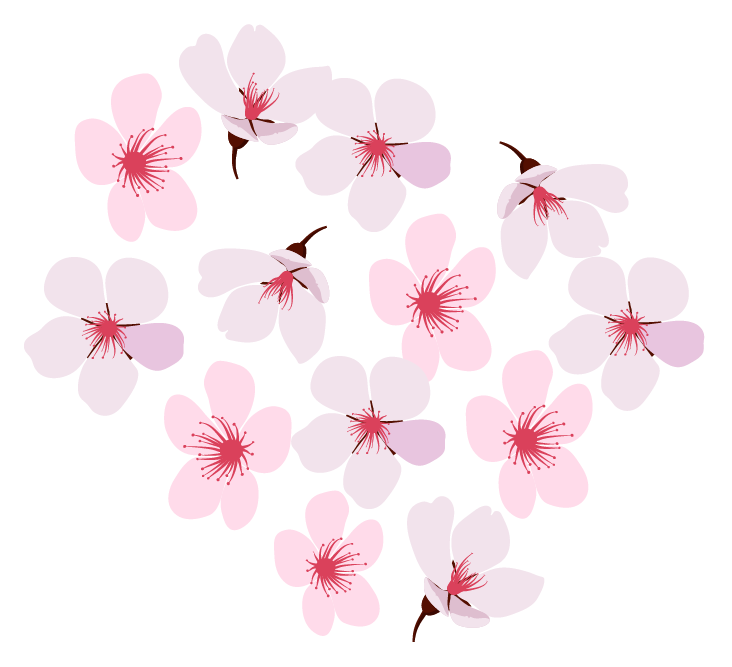Ans: The number of flowers in a bunch is 13

The number of petals in each flower are 5.

Number of petals in 13 flowers

$= 13 \times 5 = 65$

\begin{align} \,\,\,50 \\ \underline { + 15} \\ \,\,\,65 \\ \end{align}

b) A book has 64 pages. What will be the total number of pages in 8 such books?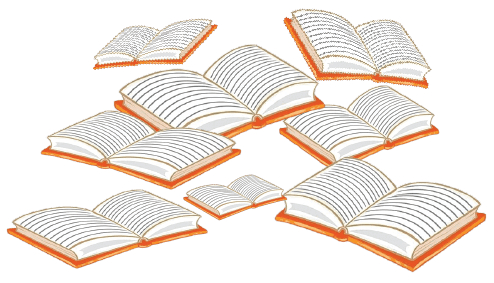Ans:

The total number of books is 8.

The number of pages in each book is 64.

Number of pages in 8 books

$= 8 \times 64 = 512$

\begin{align} 480 \\ \underline { + 32} \\ \,\,\,65 \\ \end{align}

c) Students stand in rows in the assembly. There are six rows of students. Each row has 17 students. How many students are there?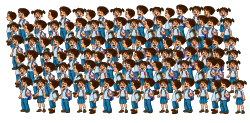Ans: Total number of rows in assembly is 6.

The number of students in each row is 17.

Number of students in 6 rows

$= 6 \times 17 = 102$

\begin{align} \,\,\,60 \\ \underline { + 42} \\ \,\,102 \\ \end{align}

d) A design has 3 flowers in it. A piece of cloth has 17 such designs. How many flowers will be on the cloth?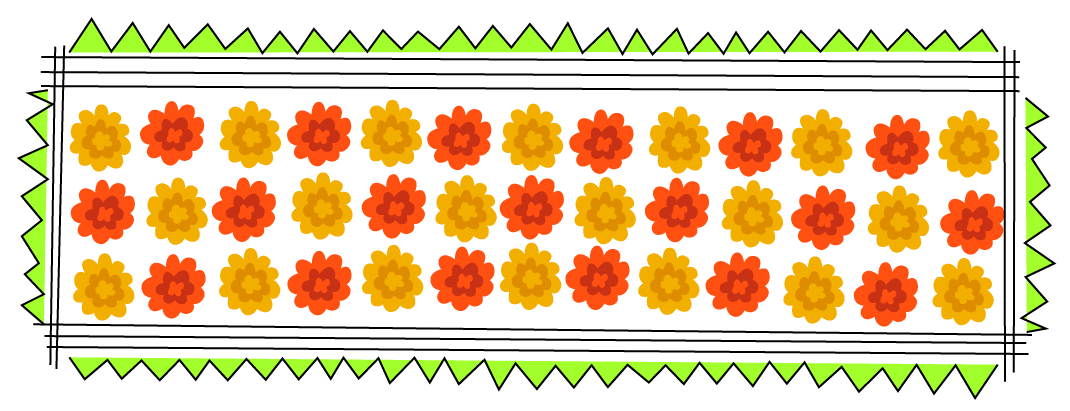Ans: The number of designs in a cloth is 17.

The number of flowers in each design is 3.

Number of flowers in 17 designs

$= 17 \times 3 = 51.$

\begin{align} \,\,\,30 \\ \underline { + 21} \\ \,\,\,51 \\ \end{align}

26. Practice Time First guess the answer and then check it by calculating:

a) $42 \times 23 = \_\_\_\_$

Ans: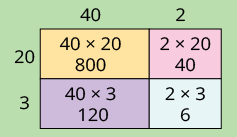Adding the number in the boxes:

\begin{align} 8\,0\,0 \\ 1\,2\,0 \\ \,\,4\,0 \\ \underline {\, + 6} \\ 966 \\ \end{align}

Hence, $42 \times 23 = 966$

b) $51 \times 13 = \_\_\_\_$

Ans: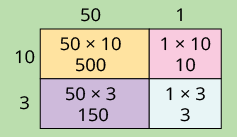Adding the number in the boxes:

\begin{align} 5\,0\,0 \\ 1\,5\,0 \\ \,\,\,1\,0 \\ \underline { + \,\,3} \\ 663 \\ \end{align}

Hence, $51 \times 13 = 663$

c) $25 \times 36 = \_\_\_\_$

Ans: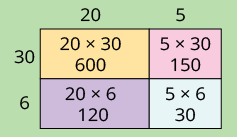Adding the number in the boxes:

\begin{align} 6\,0\,0 \\ 1\,2\,0 \\ 1\,5\,0 \\ \underline { + 3\,0} \\ 900 \\ \end{align}

Hence, $25 \times 36 = 900$

d) $73 \times 11 = \_\_\_\_$

Ans: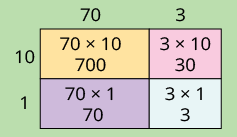Adding the number in the boxes:

\begin{align} 7\,0\,0 \\ \,\,7\,0 \\ \,\,3\,0 \\ \underline { + \,\,0} \\ 803 \\ \end{align}

Hence, $73 \times 11 = 803$

e) $54 \times 12 = \_\_\_\_$

Ans: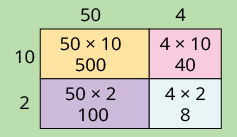Adding the number in the boxes:

\begin{align} 5\,0\,0 \\ 1\,0\,0 \\ \,\,4\,0 \\ \underline { + \,\,8} \\ 648 \\ \end{align}

Hence, $54 \times 12 = 648$

f) $12 \times 14 = \_\_\_\_$

Ans: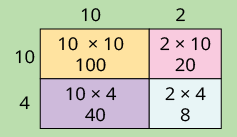Adding the number in the boxes:

\begin{align} 1\,0\,0 \\ \,\,40 \\ \,\,20 \\ \underline { + \,\,8} \\ 168 \\ \end{align}

Hence, $12 \times 14 = 168$

27. Multiplication Patterns

 $9 \times 1 = 9$ $9 \times 2 = 18$ $1 + 8 = 9$ $9 \times 3 = 27$ $2 + 7 = 9$ $9 \times 4 = 36$ $3 + 6 = 9$ $9 \times 5 = 45$ $4 + 5 = 9$ $9 \times \_\_\_ = \_\_\_$ $\_\_\_ + \_\_\_ = \_\_\_$ $9 \times \_\_\_ = \_\_\_$ $\_\_\_ + \_\_\_ = \_\_\_$ $9 \times 8 = \_\_\_$ $\_\_\_ + \_\_\_ = \_\_\_$

Did you see the pattern in the 9 times table? What numbers are adding up to 9?

Ans: The multiplication pattern is explained in the table given below.

One’s digit of all the numbers is in descending order such that 8, 7, 6, 5, 4, 3, 2, 1.

The tens digit are the numbers in ascending order such that 1, 2, 3, 4, 5, 6, 7, 8.

One’s digit number and tens digit number add to 9

 $9 \times 1 = 9$ $9 \times 2 = 18$ $1 + 8 = 9$ $9 \times 3 = 27$ $2 + 7 = 9$ $9 \times 4 = 36$ $3 + 6 = 9$ $9 \times 5 = 45$ $4 + 5 = 9$ $9 \times 6 = 54$ $5 + 4 = 9$ $9 \times 7 = 63$ $6 + 3 = 9$ $9 \times 8 = 72$ $7 + 2 = 9$

28. Complete the Grid by Multiplying the Numbers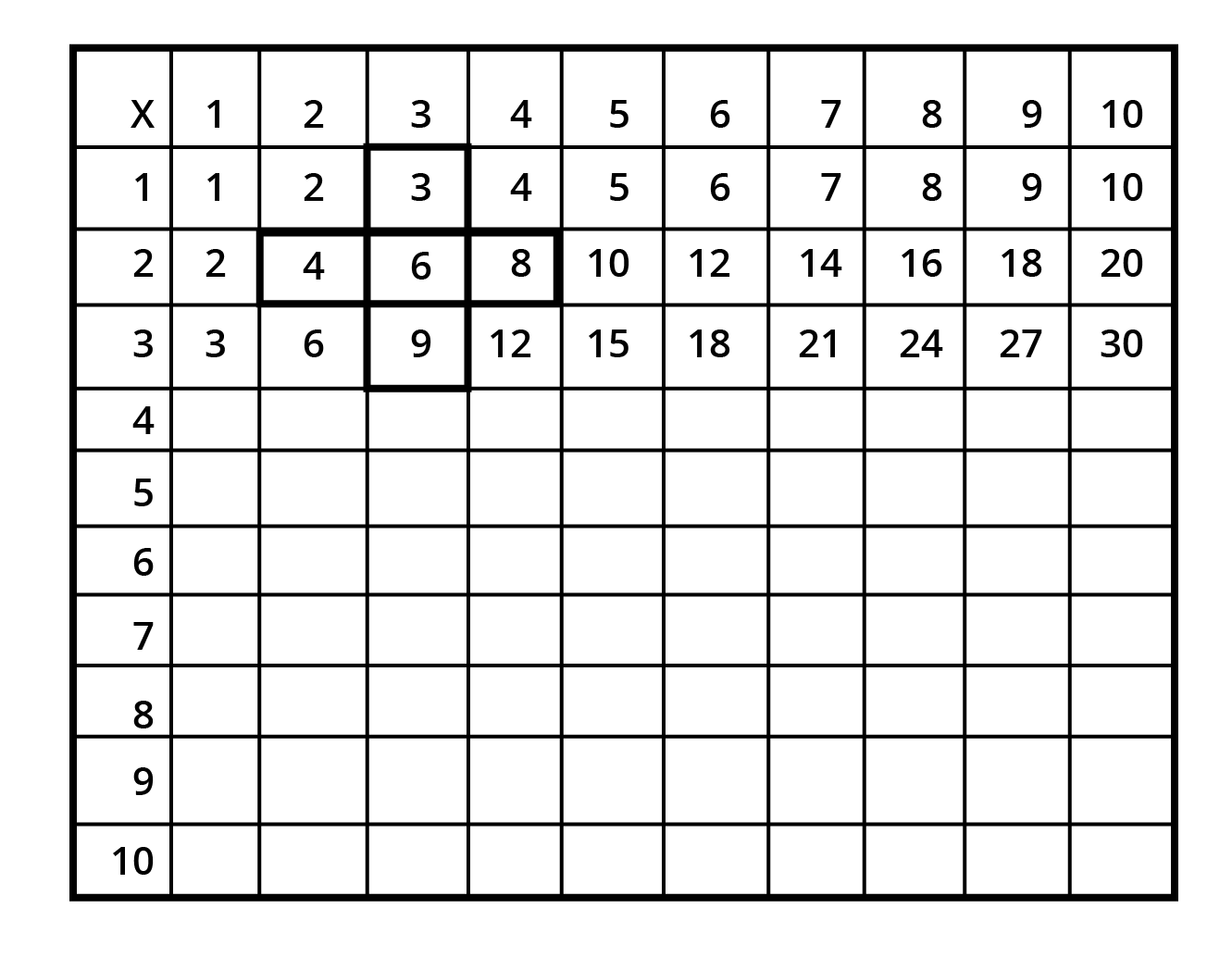Ans: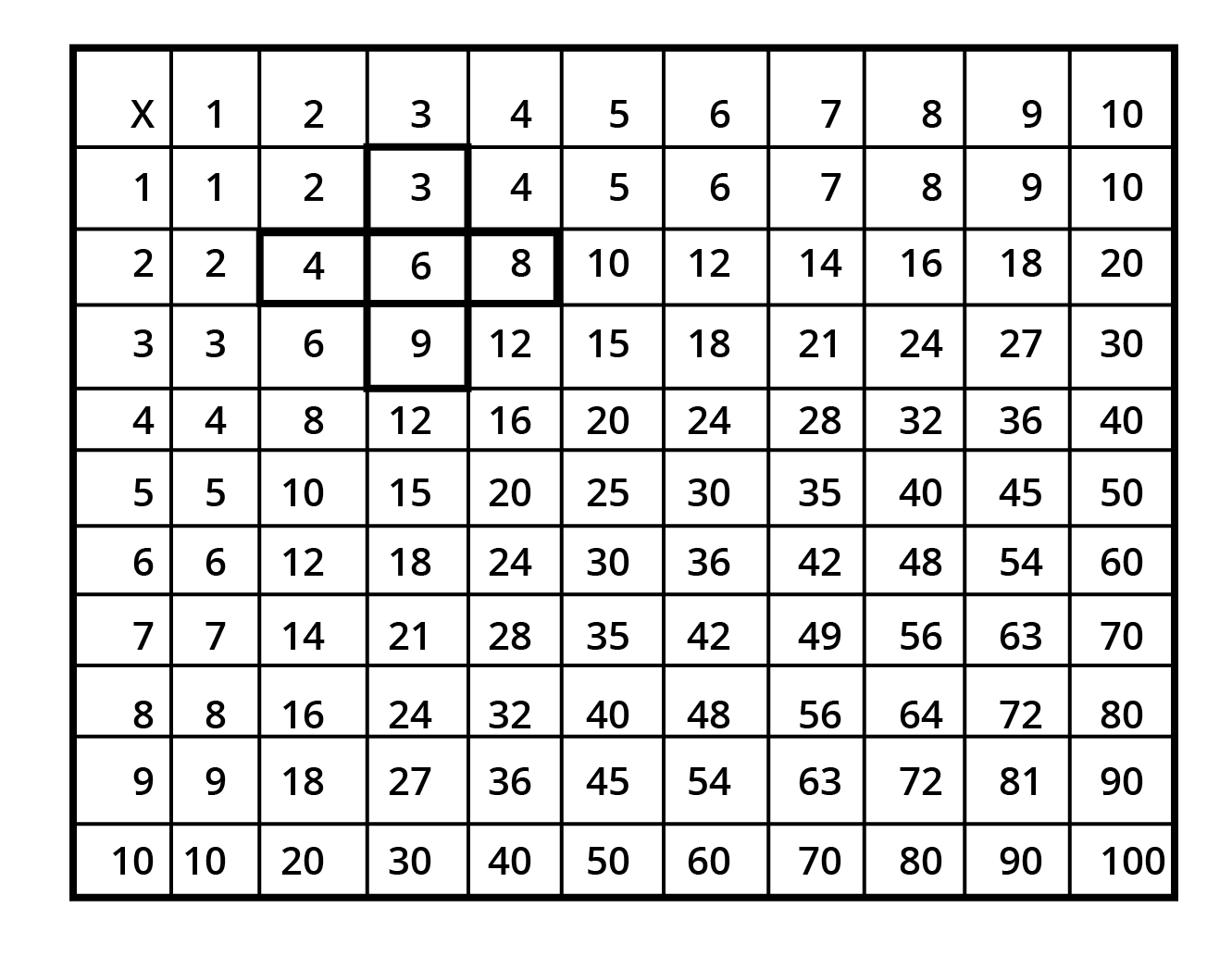29. Mark the numbers 1-10 in the same grid in one colour.

a) Mark the numbers 12-20 in another colour.

b) Similarly mark 21-30 in a third colour. Do you see any colour pattern?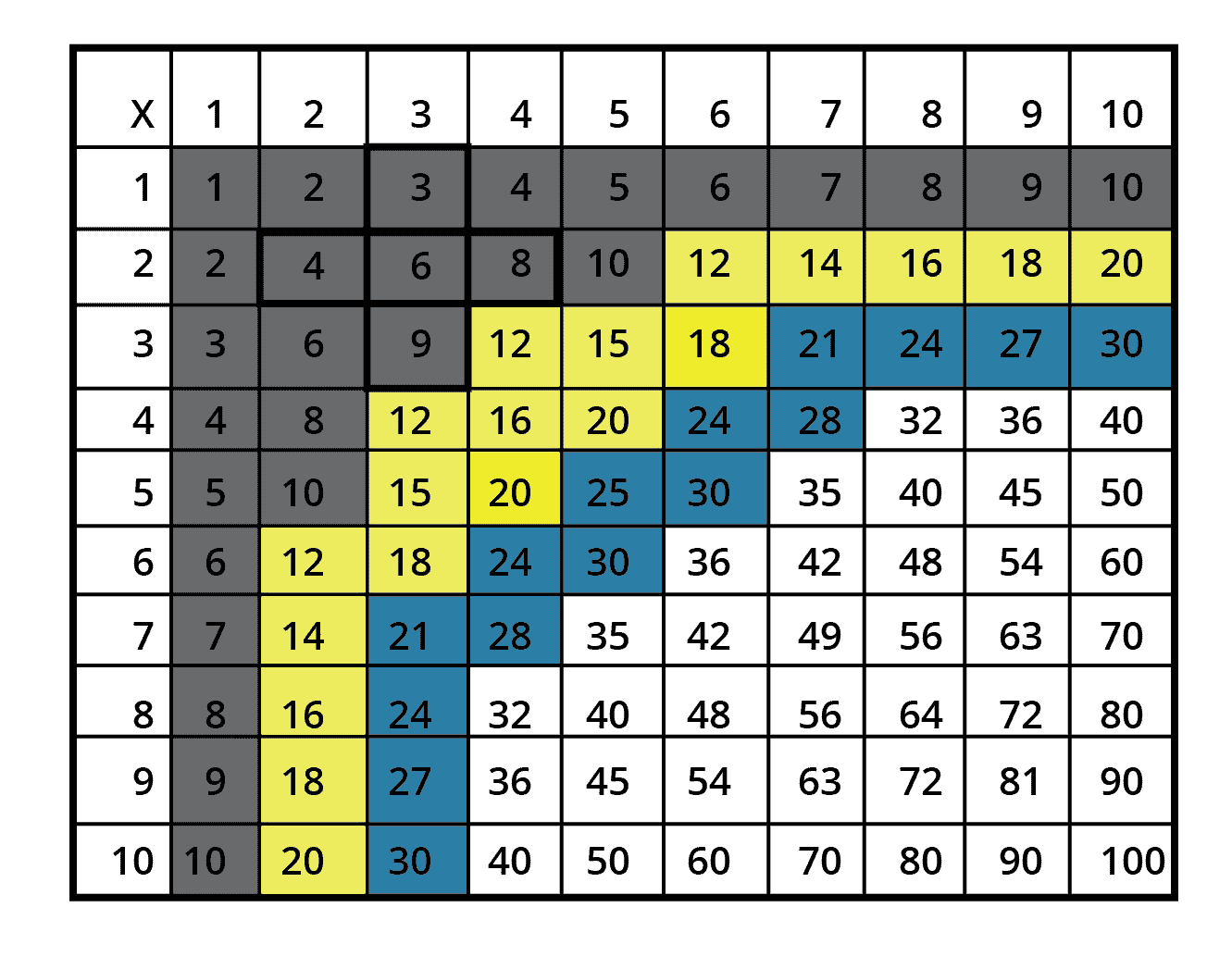Ans: No, we cannot see any colour pattern.

30. Fill this Space with Your Favourite Multiplication Table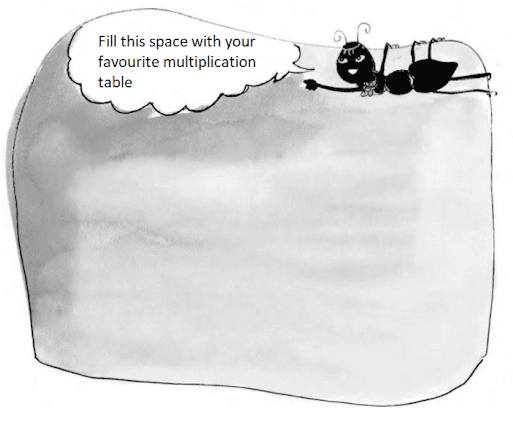Ans:

$2 \times 1 = 2$

$2 \times 2 = 4$

$2 \times 3 = 6$

$2 \times 4 = 8$

$2 \times 5 = 10$

$2 \times 6 = 12$

$2 \times 7 = 14$

$2 \times 8 = 16$

$2 \times 9 = 18$

$2 \times 10 = 20$

The answer may vary from student to student. You can choose any of your favorite tables.

## NCERT Solutions Class 3 Maths Chapter 9 – Free PDF Download

Mathematics induces reasoning, creativity, abstract or spatial thinking, critical thinking, problem-solving ability, and even effective communication skills. The syllabus of NCERT Class 3 Mathematics covers some important topics. Vedantu experts have formulated high-quality solutions for Class 3 Maths Chapter 9 How Many Times. Download free PDF for Class 3 Chapter 9 Solution for NCERT Maths to gain a conceptual learning experience.

The Class 3 Maths Chapter 9 How Many Times focuses on teaching tables, multiplications using big numbers and product patterns. Simply download NCERT Solutions for Class 3 Maths Chapter 9 How Many Times to learn and practice the concepts of multiplication. The lucid explanations help the student strengthen concepts and practice more.

### NCERT Solutions Class 3 How Many Times

Repetitive addition and evaluation of products are some of the important topics taught in this chapter. NCERT Chapter 9 How Many Times also introduces multiplication using big numbers. The fun activities and practice problems assist students to develop a conceptual understanding of multiplication. Class 3 Maths Ch 9 NCERT Solutions guides the student on a stepwise approach towards estimating the result of the operation. The following exercises have been solved under CBSE Class 3 Maths Chapter 9 Solutions.

 Maths Class 3 Chapter 9: Exercises Page 122: Leggy Animals Page 123: Find the Number without Counting Page 124-125: Practice Time Page 126: How Many Times 2? Page 127: Jump With Me Page 128: Show jumps with 4 steps Page 129: Try jumps with 7 steps Page 130-132: Stick Play Page 132-135: Practice Time Page 138-139: Practice Time Page 141: Practice Time Page 141-143: Multiplication Patterns

### Class 3 Maths Chapter-Wise Marks Weightage

In CBSE Class 3 syllabus all chapters are crucial for sharpening quantitative aptitude. However, the chapter-wise weightage of the NCERT Class 3 Maths Syllabus is given as below:

• Where to look from? - 4

• Fun with Numbers – 12

• Give and Take – 8

• Long and Short – 5

• Shapes and Designs – 10

• Fun with Give and Take – 7

• Time Goes on – 8

• Who is Heavier – 5

• How many times? – 10

• Play with Patterns – 8

• Jugs and Mugs – 7

• Can We Share? – 5

• Smart Charts – 6

• Rupees and Paise – 5

From the above division of marks weightage, it is clear that you need to have a solid understanding of numbers so that you can learn the rest of the lessons.

### Benefits of NCERT Solutions of Class 3 Maths Chapter 9

NCERT Solutions Class 3 Maths How Many Times have been prepared as per the CBSE norms to help students secure good marks in the examinations. The following are the benefits of referring to Vedantu’s solution.

• Step-wise concept building is given a priority while preparing these solutions.

• The high quality, error-free and precise reasoning help students to learn the concepts fast.

• Proficient subject-matter experts provide strong concepts, tips, and chapter insights.

• Important definitions and formulas are included in the Solutions for a better understanding of the solutions.

• A step-by-step problem-solving approach facilitates easy understanding.

The NCERT Solutions for Class 3 Maths Chapter 9 How Many Times are prepared in an easy-to-understand manner.

### Q1. What is the Importance of Maths Class 3 Chapter 9 in the Curriculum?

Ans: The chapter teaches the number of days in a week month and a year. It gives a comprehensive idea about time and calendar. The four seasons and the temperature changes associated with them are also discussed.

### Q2. How Many Legs Does a Cockroach Have? So, Altogether, How Many Legs Do 5 Cockroaches have?

Ans: A cockroach has 6 legs.

Therefore, 5 cockroaches have = 6 + 6+ 6+ 6+6 i.e. 5 times 6 or 6X5 = 30

5 cockroaches have 30 legs, in total.

### Q3. A Crab Has 10 Legs. How Many Legs Do 6 Crabs Have?

Ans: 6 crabs have = 10+10+10+10+10+10 i.e. 6 times 10 or 6X10 = 60.

Therefore, 6 crabs have 60 legs.

Q4. 4 X 20 is _ Times _ or _ + _ + _ + _ =?

Ans: 80

Q5. 10 Times 10 is How Much?

Ans: 10 times 10 = 10+10+10+10+10+10+10+10+10+10 i.e. 10X10 = 100.

Vedantu’s CBSE Class 3 Maths Chapter 9 Solutions have many such important questions which if you practise will help you understand the chapter better.

## FAQs on NCERT Solutions for Class 3 Maths Chapter 9 - How Many Times

Q1. Why is NCERT Class 3 Maths Chapter 9 Important?

Ans: The activities given in Class 3 Maths Chapter 9 How Many Times are designed to develop conceptual understanding of multiplication among students. The standard method for multiplying larger numbers is efficient, but it may be challenging for young students. The method used here builds up students’ growing sense of two-digit, and three-digit numbers. They are encouraged to estimate the result of the multiplication operation. Observing patterns in multiplication tables deepens the understanding of the number system.

Q2. How Important are the NCERT Solutions For Class 3 Maths Chapter 9?

Ans: The solutions of Class 3 Chapter 9 How Many Times are important for last-minute revision and concept building of multiplication. On Vedantu you can get free PDF files for solutions Ch 9 Class 3 Maths and study materials like FREE LIVE MASTER- Classes, and FREE Conceptual Videos to boost your confidence in the topic.

Ans: Yes, all the NCERT Solutions for Class 3 Mathematics Chapter 9  provided by Vedantu are completely free to download. The solutions can be downloaded in a few simple steps:

• Visit the page NCERT Solutions for Class 3 Mathematics Chapter 9.

• The link will take you to the NCERT Solutions for Class 3 Mathematics Chapter 9.

Q4. Are the NCERT Solutions provided by Vedantu explained in detail?

Ans: All the solutions provided by Vedantu are framed by our expert teachers in a step-by-step and detailed manner. Vedantu’s professionals make sure that the solutions are easy to understand for Class 3 students. The answers are also explained by relevant figures and diagrams wherever required. These solutions are perfectly suited for young learners and help them to grasp the concepts quickly and efficiently.

Q5. Is Class 3 Mathematics Chapter 9 an important chapter?

Ans: NCERT’s Class 3 Chapter 9 “How Many Times” carries a total weightage of 10 marks. Hence, it is indeed an important chapter concerning scoring well in examinations. In addition to that, the chapter teaches concepts that will be beneficial for the students in their upcoming academic years. It also lays down strong foundations for basic mathematical operations. Thus, students should practice the concepts of the chapter well.

Q6. What does Chapter 9, “How many times,” aim to teach the students?

Ans: Chapter 9, “How many times,” deals with the important concept of multiplication and repeated addition. With the help of this chapter, students will be able to understand the link between addition and multiplication. Thus, Chapter 9 teaches the students significant mathematical operations that will lay the foundation for future know-how. With the help of several practice questions and activities, Chapter 9 aims to strengthen and perfect the concept of multiplication.

Q7. How to make Class 3 students effectively understand the concept of Multiplication and Repeated Addition?

Ans: The fundamentals of multiplication and repeated addition are extremely important in laying the foundations of basic mathematics. So to excel in this chapter:

• Students need to understand the basic operation of multiplication well. They can make use of Vedantu’s study material available on the Vedantu app also for the same.

• For any mathematical concept, consistent practice is the key. Students need to practice the questions of their textbook persistently. They can refer to Vedantu’s NCERT Solutions of Class 3 Maths Chapter.